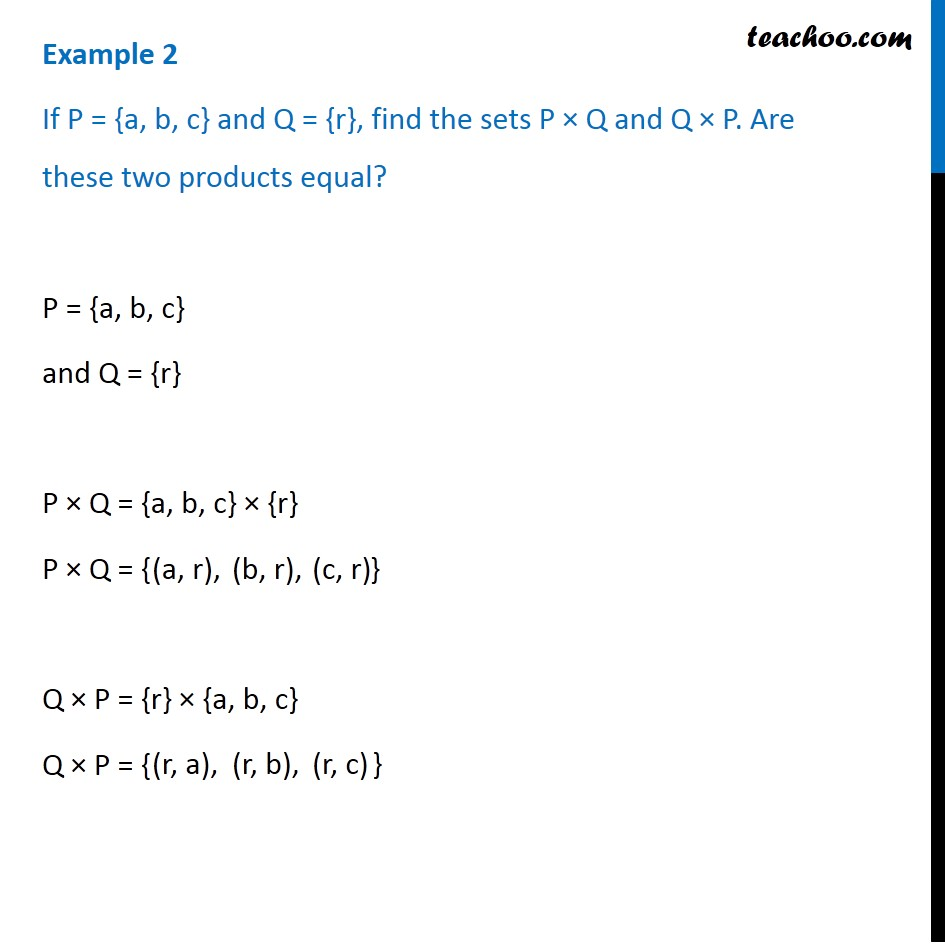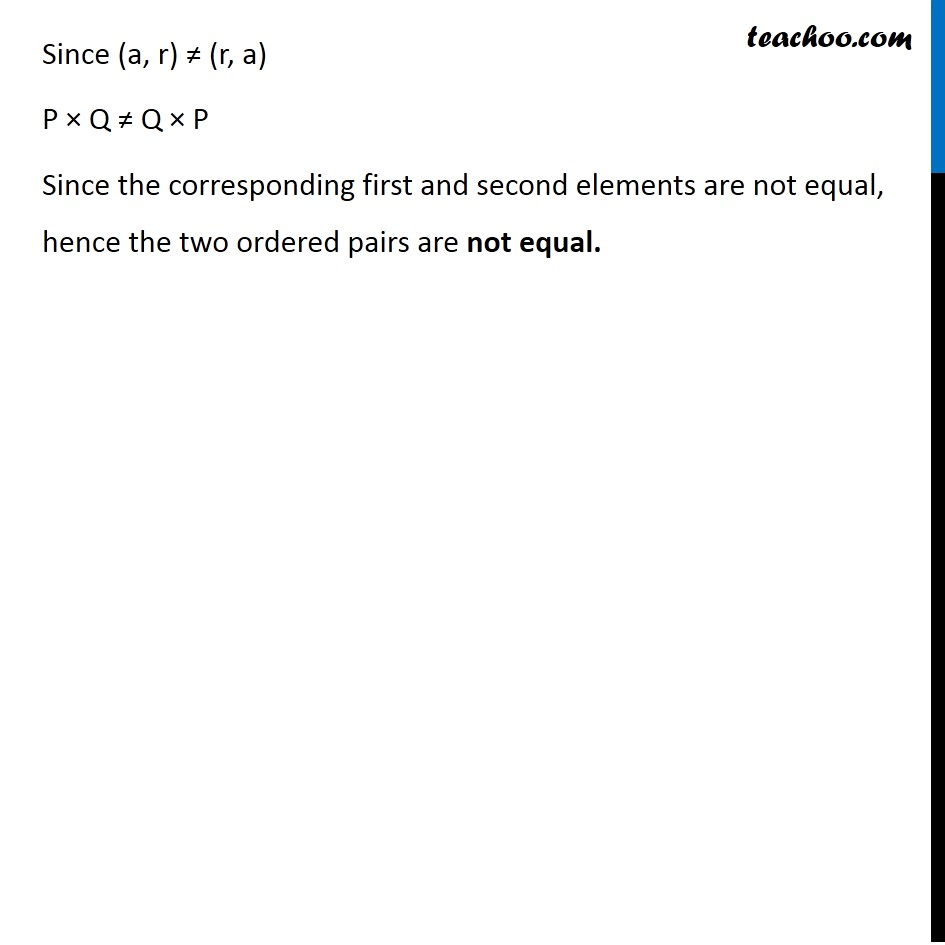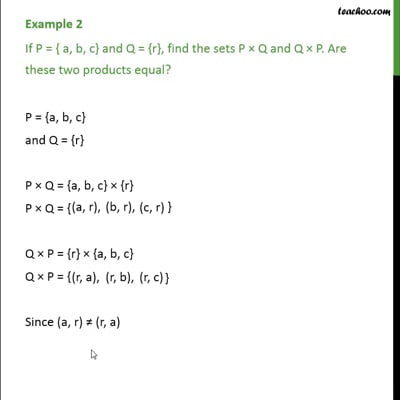Examples

Chapter 2 Class 11 Relations and Functions (Term 1)
Serial order wiseThis video is only available for Teachoo black users

### Transcript

Example 2 If P = {a, b, c} and Q = {r}, find the sets P × Q and Q × P. Are these two products equal? P = {a, b, c} and Q = {r} P × Q = {a, b, c} × {r} P × Q = { Q × P = {r} × {a, b, c} Q × P = { (a, r), (b, r), (r, a), (r, b), Since (a, r) ≠ (r, a) P × Q ≠ Q × P Since the corresponding first and second elements are not equal, hence the two ordered pairs are not equal.